# 一、数据清洗

``````import pandas as pd
import matplotlib.pyplot as plt
import jieba
from pyecharts.charts import WordCloud
from pyecharts import options as opts
import re
print(te)``````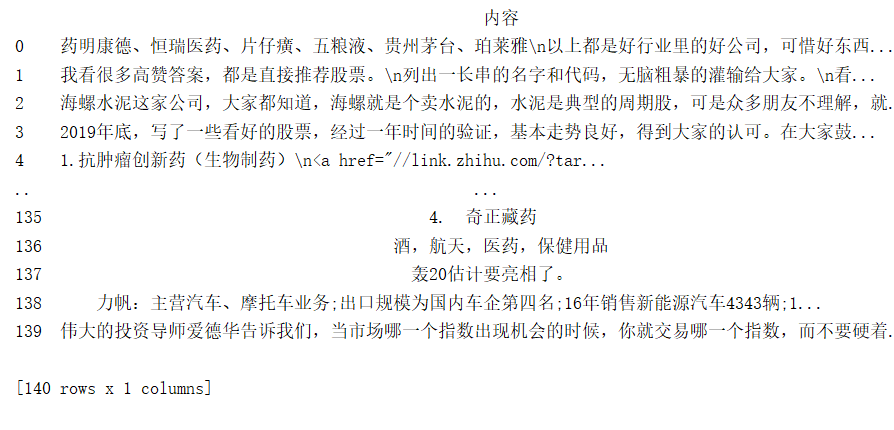``````ck0=[]#新建一个新的list
for i in range(len(te)):#循环
te1=te.内容.iloc[i]#读取每一行的数据
x=re.split('、|,|; |\*|\n',te1)#通过、,;\等分隔开来
for i in x:#原来的一个单元一个字符串就变成了一个单元多个字符串
if 1<len(i) <= 4 and type(i)==str and i.isdigit()==False:
#考虑到答案中大多数都是股票名称，一般股票名称占位4个长度，简称小于至少大于1个长度
#所以判断小于等于4个，大于1个的时候，进行添加
#同时将是字符串的数字进行排除
ck0.append(i)
print(len(ck0))
#一共采取787条信息
#将list转化为dataframe
df = pd.DataFrame(ck0, columns=['infomation'])
#print(df)``````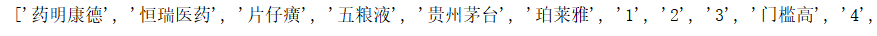list格式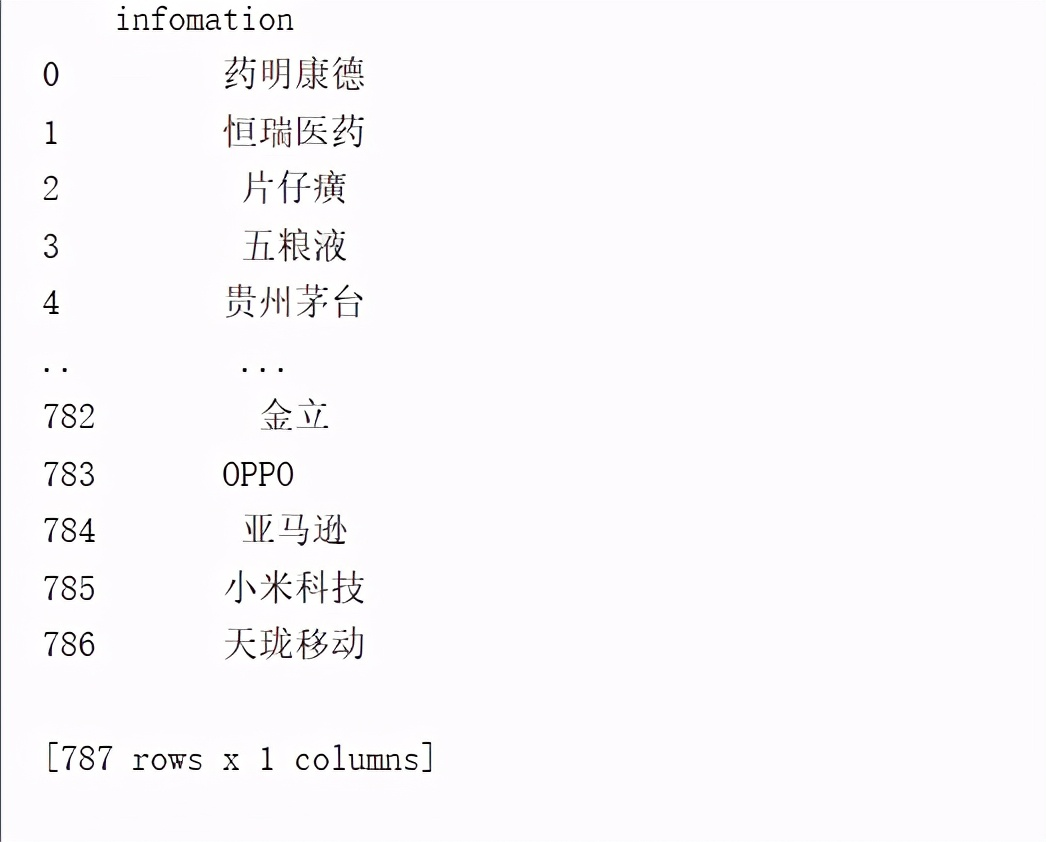dataframe数据信息787条

# 二、简单统计

``````#由于只是series，所以增加一列方便统计，计算
df['count_num']=0
#通过groupy和agg统计
df01=df.groupby('infomation').agg({'count_num':'count'})
#降序处理
df01=df01.sort_values(by='infomation',ascending=False)
df01[15:40].plot(kind='bar')
plt.xticks(rotation=45)
plt.title('关键字展示')
plt.show()``````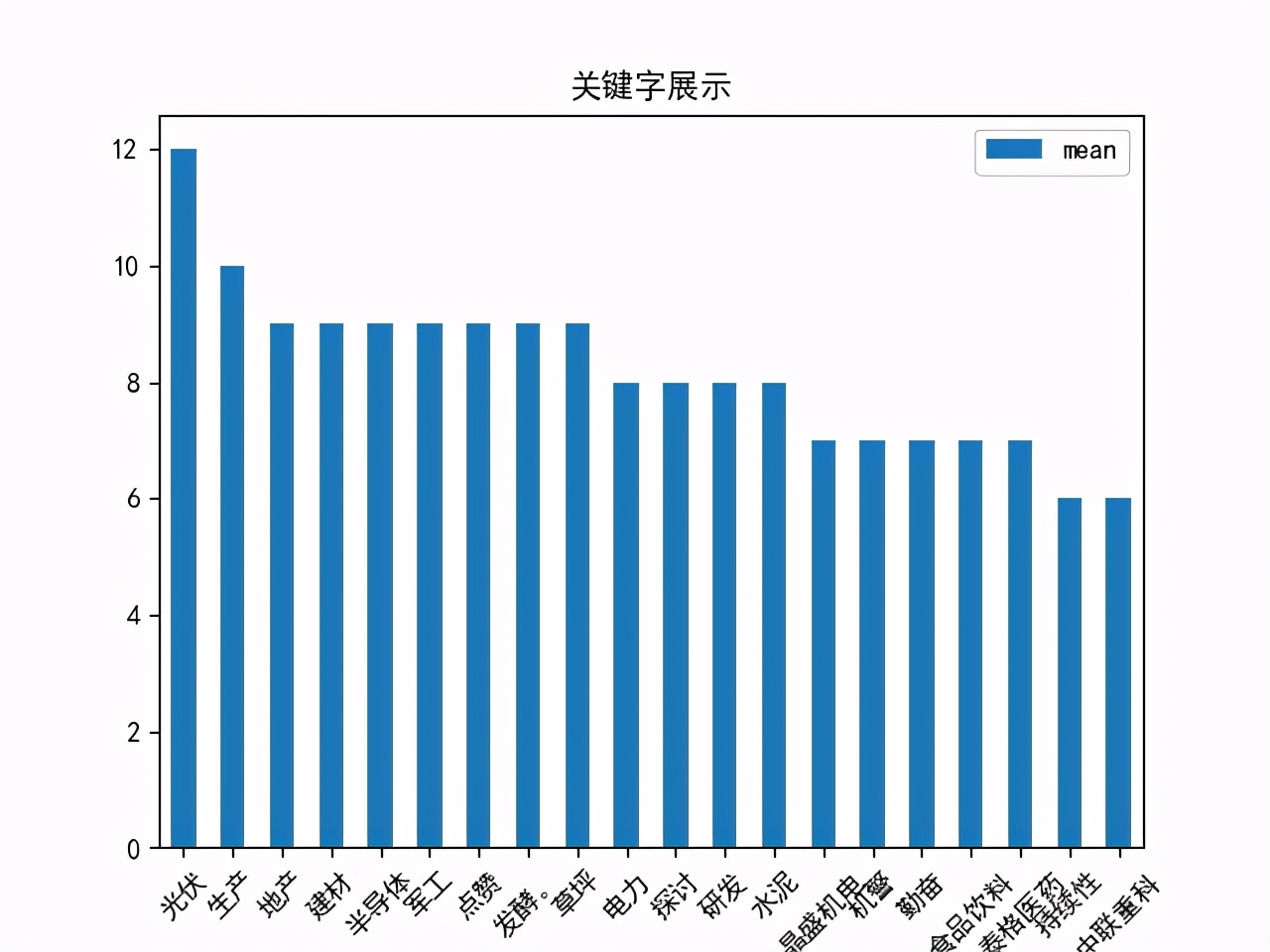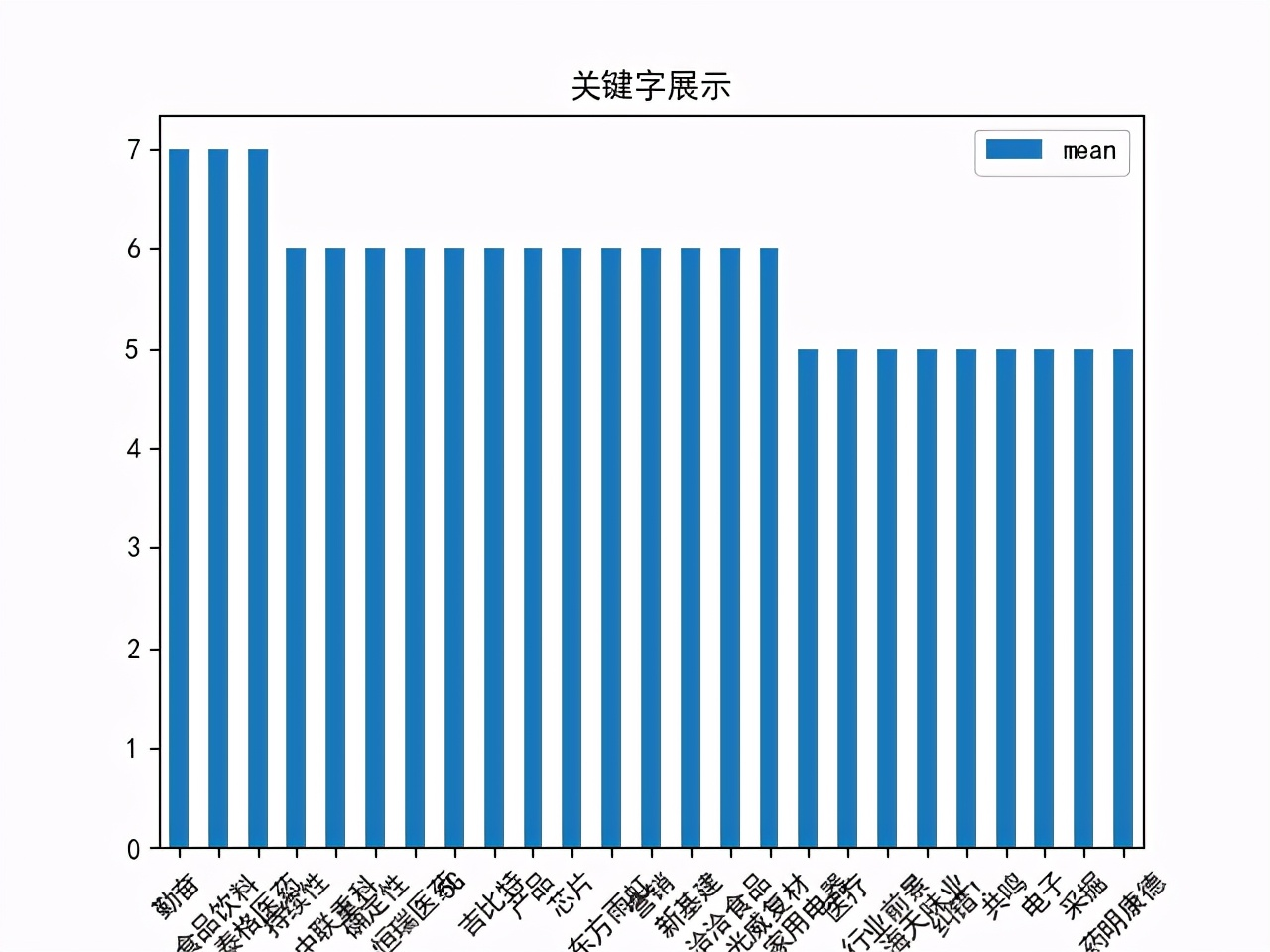# 三、结论和提示：

• 由于笔者对于jieba等库不了解，所以只能进行简单处理，由于答题的作者书写习惯不同，笔者能力有限，只能尽可能保障书写完整的股票名称进行统计，所以这个统计分析的效果只能说是一般，只能作为一点点参考，同时关于《2021年接来下哪些基金会大涨？》笔者就不去统计了，因为书写的差异性更大，更不好统计。
• 这只是笔者自己的一个Python学习小思路，不代表投资建议和看法，请读者谨慎对待。
• 未来的样本和关注度笔者将尽量关注市场的一些看法和行情分析，通过更加精准的看法分析，得出市场变化。
• 提高对于Python更高级的使用方法才是。